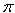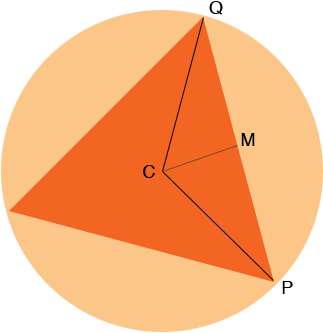SEARCH HOMEMath Central Quandaries & QueriesWhat is the area of an equilateral triangle inscribed in a circle whose circumference is 6 pi?? PLEASE HELPHi Caitlin,

The circumference of a circle is 2r and your circle has a circumference of 6. Thus

6= 2r

and therefore r = 3.

In the diagram C is the centre of the circle and M is the midpoint of PQ. The area of the inscribed circle is 3 time the area of triangle PQC.The area of a triangle is half the base times the height and hence the area of triangle PQC is |MQ||MC|.

Since the circle has radius 3, |QC| = 3. Also the inscribed triangle is equilateral and thus each of its angles measures 60 degrees. Thus the measure of angle MQC is 30 degrees and angle CMQ is a right angle so the measure of angle QCM is 60 degrees. Can you now find the lengths |MC| and |MQ|?

PennyMath Central is supported by the University of Regina and The Pacific Institute for the Mathematical Sciences.